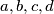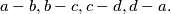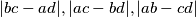### IMO Shortlist 1996 problem N1

Kvaliteta:
Avg: 2,0
Težina:
Avg: 4,5
Four integers are marked on a circle. On each step we simultaneously replace each number by the difference between this number and next number on the circle, moving in a clockwise direction; that is, the numbers$a,b,c,d$ are replaced by$a-b,b-c,c-d,d-a.$ Is it possible after 1996 such to have numbers$a,b,c,d$ such the numbers$|bc-ad|, |ac - bd|, |ab - cd|$ are primes?
Izvor: Međunarodna matematička olimpijada, shortlist 1996